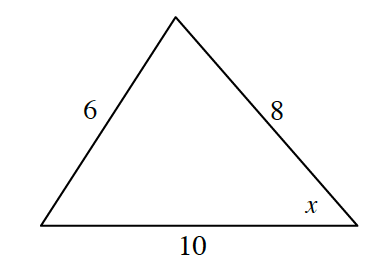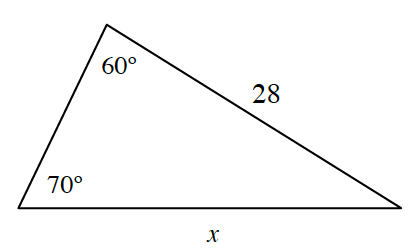### Home > PC3 > Chapter 6 > Lesson 6.2.3 > Problem6-104

6-104.

Solve for $x$ and calculate the area of each triangle below.

Label all of the sides and angles first. Standard labeling is capital letters for angles.
The side opposite the angle is the lower case letter of the angle.

$c^2=a^2+b^2-2ab\cos(C)$

$\frac{\sin(A)}{a}=\frac{\sin(B)}{b}=\frac{\sin(C)}{c}$

1.1.